# TensorFlow从1到2（十三）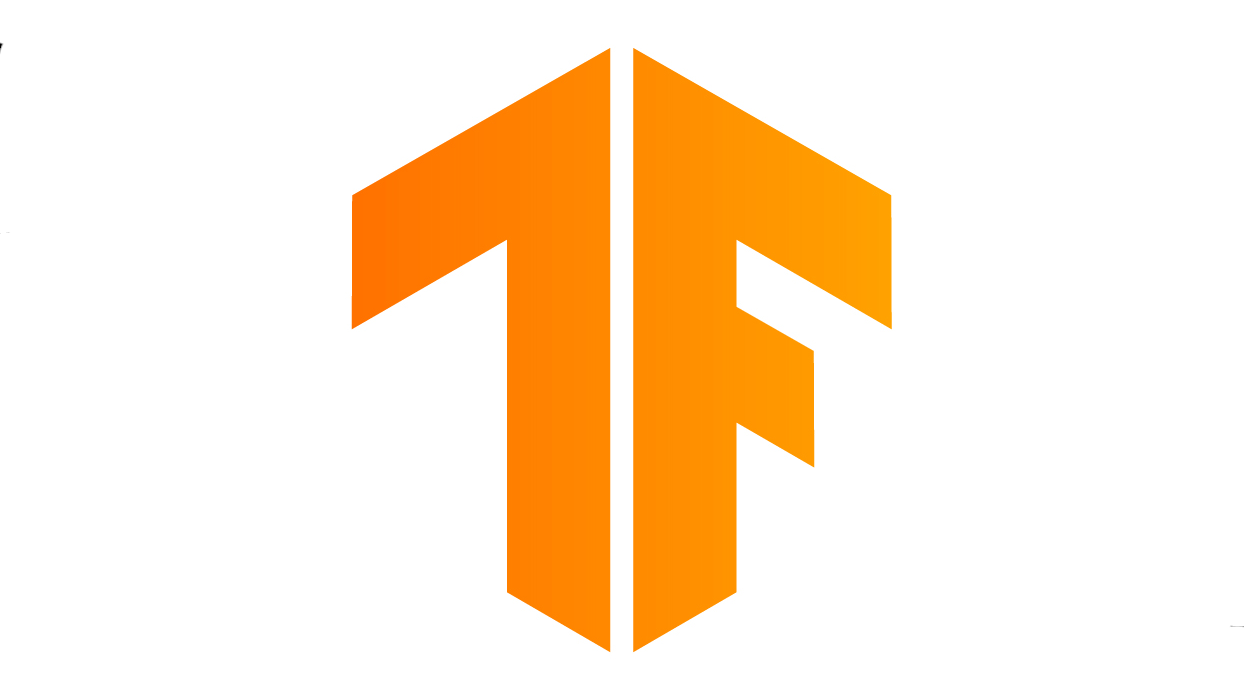#### 风格迁移

《从锅炉工到AI专家(8)》中我们介绍了一个“图片风格迁移”的例子。因为所引用的作品中使用了TensorFlow 1.x的代码，算法也相对复杂，所以文中没有仔细介绍风格迁移的原理。

“风格迁移”指的是将艺术作品的笔触、技法等表现出来的视觉效果，应用在普通照片上，使得所生成的图片，类似使用同样笔触、技法所绘制完成，但内容跟照片相同的“伪画作”。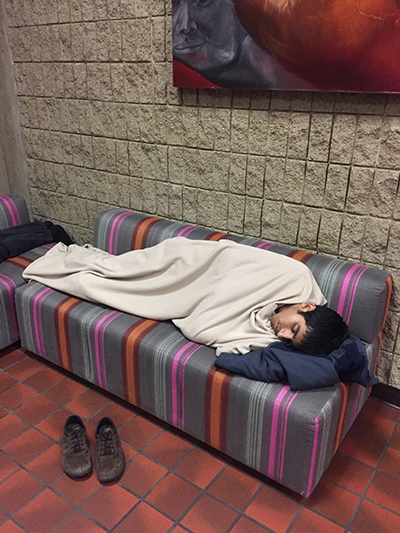接着不陌生，著名大作《星空》：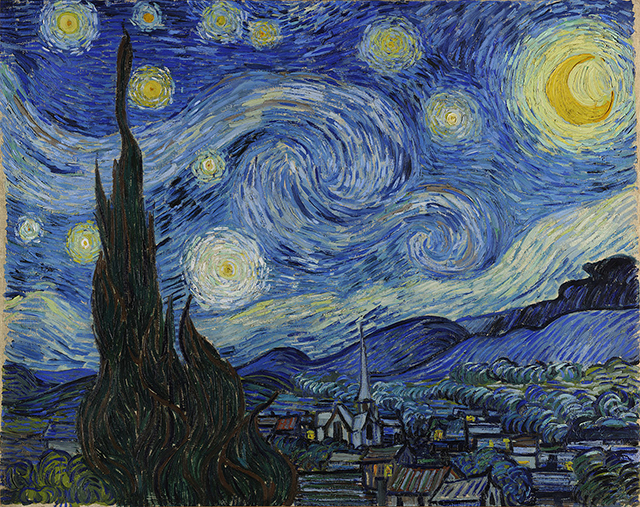（请将以上两图保存至工作目录，不要修改文件名，我们稍晚的代码中会用到。）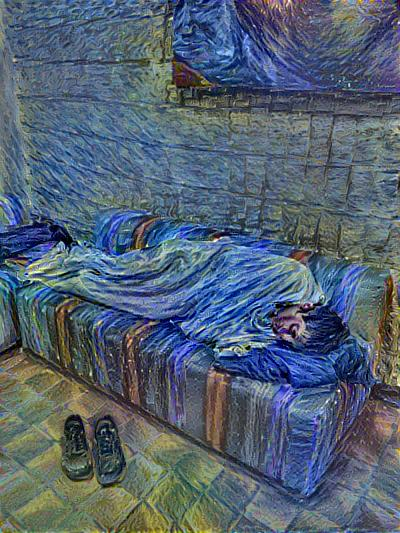#### 基本原理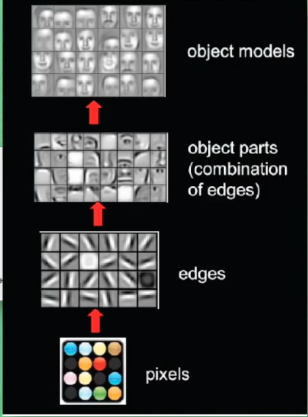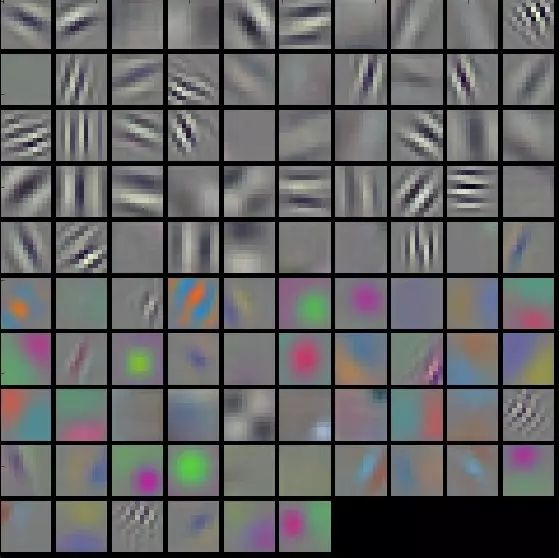最终研究成果确立了卷积神经网络进行图片迁移的两大基础算法：

• 在神经网络中，确定的抽取某些层代表内容的数字描述，以及另外一些层代表风格的数字描述。
• 多个层的输出数据，通过公式的计算，拟合到同输入图像相同的色域空间。这个公式即能用于代价函数中原始风格同目标风格之间的对比，也可以变形后通过组合多个风格层，生成新的目标图片。

``````# 定义最能代表内容特征的网络层
content_layers = ['block5_conv2']

# 定义最能代表风格特征的网络层
style_layers = ['block1_conv1',
'block2_conv1',
'block3_conv1',
'block4_conv1',
'block5_conv1']
``````

``````    ...
# 建立无需分类结果的vgg网络
vgg = tf.keras.applications.VGG19(include_top=False, weights='imagenet')

# 显示vgg中所有层的名称
print()
for layer in vgg.layers:
print(layer.name)
...
``````

• 生成图片的风格层输出，同艺术图片的风格层输出差异最小
• 生成图片的内容层输出，同原始照片的内容层输出差异最小

#### 源代码

``````#!/usr/bin/env python3

from __future__ import absolute_import, division, print_function, unicode_literals

import tensorflow as tf
import matplotlib.pyplot as plt
import matplotlib as mpl
import numpy as np
import time
import functools
import time
from PIL import Image

# 设置绘图窗口参数，用于图片显示
mpl.rcParams['figure.figsize'] = (13, 10)
mpl.rcParams['axes.grid'] = False

# 获取下载后本地图片的路径，content_path是真实照片，style_path是艺术品风格图片
content_path = "1-content.jpg"
style_path = "1-style.jpg"

# 读取一张图片，并做预处理
max_dim = 512
# 读取二进制文件
# 做JPEG解码，这时候得到宽x高x色深矩阵，数字0-255
img = tf.image.decode_jpeg(img)
# 类型从int转换到32位浮点，数值范围0-1
img = tf.image.convert_image_dtype(img, tf.float32)
# 减掉最后色深一维，获取到的相当于图片尺寸（整数），转为浮点
shape = tf.cast(tf.shape(img)[:-1], tf.float32)
# 获取图片长端
long = max(shape)
# 以长端为比例缩放，让图片成为512x???
scale = max_dim/long
new_shape = tf.cast(shape*scale, tf.int32)
# 实际缩放图片
img = tf.image.resize(img, new_shape)
# 再扩展一维，成为图片数字中的一张图片（1，长，宽，色深）
img = img[tf.newaxis, :]
return img

# 读入两张图片

############################################################
# 定义最能代表内容特征的网络层
content_layers = ['block5_conv2']

# 定义最能代表风格特征的网络层
style_layers = ['block1_conv1',
'block2_conv1',
'block3_conv1',
'block4_conv1',
'block5_conv1']
# 神经网络层的数量
num_content_layers = len(content_layers)
num_style_layers = len(style_layers)

# 定义一个工具函数，帮助建立得到特定中间层输出结果的新模型
def vgg_layers(layer_names):
""" Creates a vgg model that returns a list of intermediate output values."""
# 定义使用ImageNet数据训练的vgg19网络
vgg = tf.keras.applications.VGG19(include_top=False, weights='imagenet')
# 已经经过了训练，所以锁定各项参数避免再次训练
vgg.trainable = False
# 获取所需层的输出结果
outputs = [vgg.get_layer(name).output for name in layer_names]
# 最终返回结果是一个模型，输入是图片，输出为所需的中间层输出
model = tf.keras.Model([vgg.input], outputs)
return model

# 定义函数计算风格矩阵，这实际是由抽取出来的5个网络层的输出计算得来的
def gram_matrix(input_tensor):
result = tf.linalg.einsum('bijc,bijd->bcd', input_tensor, input_tensor)
input_shape = tf.shape(input_tensor)
num_locations = tf.cast(input_shape*input_shape, tf.float32)
return result/(num_locations)

# 自定义keras模型
class StyleContentModel(tf.keras.models.Model):
def __init__(self, style_layers, content_layers):
super(StyleContentModel, self).__init__()
# 自己的vgg模型，包含上面所列的风格抽取层和内容抽取层
self.vgg = vgg_layers(style_layers + content_layers)
self.style_layers = style_layers
self.content_layers = content_layers
self.num_style_layers = len(style_layers)
# vgg各层参数锁定不再参数训练
self.vgg.trainable = False

def call(self, input):
# 输入的图片是0-1范围浮点，转换到0-255以符合vgg要求
input = input*255.0
# 对输入图片数据做预处理
preprocessed_input = tf.keras.applications.vgg19.preprocess_input(input)
# 获取风格层和内容层输出
outputs = self.vgg(preprocessed_input)
# 输出实际是一个数组，拆分为风格输出和内容输出
style_outputs, content_outputs = (
outputs[:self.num_style_layers],
outputs[self.num_style_layers:])
# 计算风格矩阵
style_outputs = [gram_matrix(style_output)
for style_output in style_outputs]

# 转换为字典
content_dict = {content_name: value
for content_name, value
in zip(self.content_layers, content_outputs)}
# 转换为字典
style_dict = {style_name: value
for style_name, value
in zip(self.style_layers, style_outputs)}
# 返回内容和风格结果
return {'content': content_dict, 'style': style_dict}

# 使用自定义模型建立一个抽取器
extractor = StyleContentModel(style_layers, content_layers)

# 设定风格特征的目标，即最终生成的图片，希望风格上尽量接近风格图片
style_targets = extractor(style_image)['style']
# 设定内容特征的目标，即最终生成的图片，希望内容上尽量接近内容图片
content_targets = extractor(content_image)['content']

# 内容图片转换为张量
image = tf.Variable(content_image)

# 截取0-1的浮点数，超范围部分被截取
def clip_0_1(image):
return tf.clip_by_value(image, clip_value_min=0.0, clip_value_max=1.0)

# 优化器
# 预定义风格和内容在最终结果中的权重值，用于在损失函数中计算总损失值
style_weight = 1e-2
content_weight = 1e4

# 损失函数
def style_content_loss(outputs):
style_outputs = outputs['style']
content_outputs = outputs['content']
# 风格损失值，就是计算方差
for name in style_outputs.keys()])
# 权重值平均到每层，计算总体风格损失值
style_loss *= style_weight/num_style_layers

# 内容损失值，也是计算方差
for name in content_outputs.keys()])
content_loss *= content_weight/num_content_layers
# 总损失值
loss = style_loss+content_loss
return loss
################################################################

# 一次训练
@tf.function()
def train_step(image):
# 抽取风格层、内容层输出
outputs = extractor(image)
# 计算损失值
loss = style_content_loss(outputs)

# 梯度下降
# 应用计算后的新参数，注意这个新值不是应用到网络
# 作为训练完成的vgg网络，其参数前面已经设定不可更改
# 这个参数实际将应用于原图
# 以求取，新图片经过网络后，损失值最小
# 更新图片，用新图片进行下次训练迭代
image.assign(clip_0_1(image))

start = time.time()
epochs = 10
steps_per_epoch = 100

step = 0
for n in range(epochs):
for m in range(steps_per_epoch):
step += 1
train_step(image)
print(".", end='')
print("")
# 每100次迭代显示一次图片
# plt.title("Train step: {}".format(step))
# plt.show()

end = time.time()
print("Total time: {:.1f}".format(end-start))

########################################
#保存结果图片
file_name = 'newart1.png'
mpl.image.imsave(file_name, image)
``````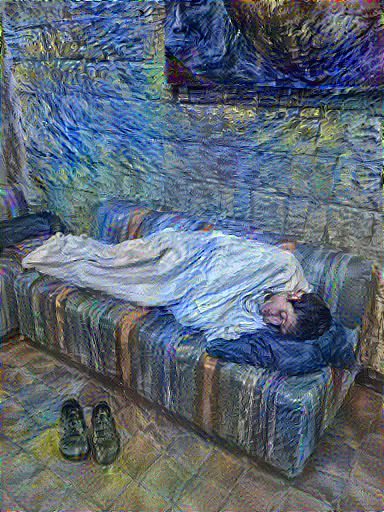看起来基本达到了设计要求，不过再仔细观察，似乎效果虽然都有了，但画面看上去有一点不干净，有很多小的噪点甚至有了干涉纹。

``````###################################################
# 计算x方向及y方向相邻像素差值，如果有高频花纹，这个值肯定会高，
# 因为相邻点相同差值接近0，区别越大，差值当然越大
def high_pass_x_y(image):
x_var = image[:, :, 1:, :] - image[:, :, :-1, :]
y_var = image[:, 1:, :, :] - image[:, :-1, :, :]

return x_var, y_var

# 计算总体变分损失
def total_variation_loss(image):
x_deltas, y_deltas = high_pass_x_y(image)
return tf.reduce_mean(x_deltas**2)+tf.reduce_mean(y_deltas**2)

# 总体变分损失值在损失值中所占权重
total_variation_weight = 1e8

# 一次训练
@tf.function()
def train_step(image):
# 抽取风格层、内容层输出
outputs = extractor(image)
# 计算损失值
loss = style_content_loss(outputs)
loss += total_variation_weight*total_variation_loss(image)

# 梯度下降
# 应用计算后的新参数，注意这个新值不是应用到网络
# 作为训练完成的vgg网络，其参数前面已经设定不可更改
# 这个参数实际将应用于原图
# 以求取，新图片经过网络后，损失值最小
# 更新图片，用新图片进行下次训练迭代
image.assign(clip_0_1(image))

# 内容图片作为逐步迭代生成的新图片，一开始当然是原图，这里是转换为张量
image = tf.Variable(content_image)

start = time.time()

# 迭代10次，每次100步训练
epochs = 10
steps = 100

step = 0
for n in range(epochs):
for m in range(steps):
step += 1
train_step(image)
print(".", end='')
print("")
end = time.time()
print("Total time: {:.1f}".format(end-start))

#保存结果图片
file_name = 'newart1.png'
mpl.image.imsave(file_name, image)
``````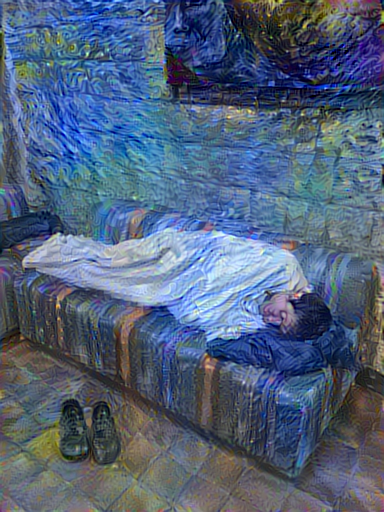效果不错吧？可以换上自己的照片还有自己心仪的艺术作品来试试。

（待续…）